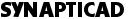# Waveform Block Equations for Timing Diagram Editors

Sine, Capacitor, Ramp and Exponential waveforms can be inserted into a waveform segment using a Waveform Equation Block. Unlike the State Label equations of the next section which append analog waveform segments onto the end of a signal, waveform equation blocks can edited after they are created. For this reason, it's generally better to use a waveform equation block rather than a State Label equation.

## Many functions have Code already written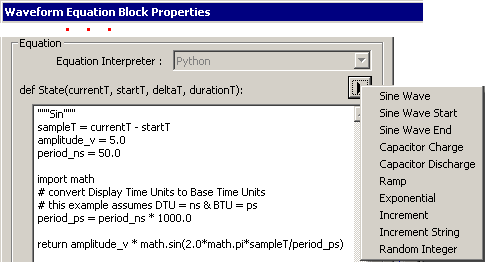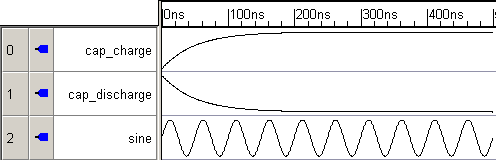## WaveForm Block Equations can appear in consecutive segments on a waveform

Checking the View > Show Waveform Block Highlights menu will draw blue boxes around all of the Waveform Equation Blocks.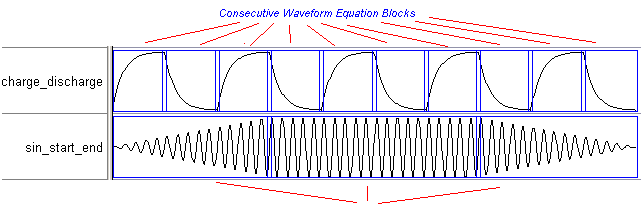## Many functions can be written by editing and combining code from the default codes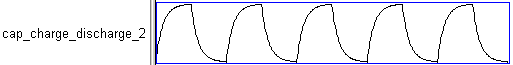```"""Cap smoothed square wave"""
period_ns = 100.0
period_ps = period_ns * 1000.0

sampleT = (currentT - startT) % (period_ps / 2)
rc_constant_ns = 10.0
amplitude_v = 5.0
# determine whether we're on the charge or discharge half of the cycle
charge = int((currentT - startT) / (period_ps / 2)) % 2 == 0

rc_constant_ps = 1000.0 * rc_constant_ns
import math
if charge:
return amplitude_v * (1.0 - math.exp(-sampleT/rc_constant_ps))
else:
return amplitude_v * math.exp(-sampleT/rc_constant_ps)

```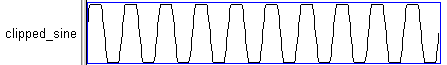```"""Clipped Sine wave"""
sampleT = currentT - startT
sin_amplitude_v = 10.0
clipping_amplitude_v = 5.0
period_ns = 50.0

import math
# convert Display Time Units to Base Time Units
# this example assumes DTU = ns & BTU = ps
period_ps = period_ns * 1000.0

sin_value = sin_amplitude_v * math.sin(2.0*math.pi*sampleT/period_ps)
return max(-clipping_amplitude_v, min(clipping_amplitude_v, sin_value))

```

## Code your own Waveform Block Equations using Python math functions```"""Fourier series for a square wave"""
# increase "k" below to get a closer approximation of
# a square wave
import math
sampleT = currentT - startT
amplitude_v = 5.0
period_ns = 50.0
k = 7

period_ps = period_ns * 1000.0

return sum([1.0/n * math.sin(2.0*math.pi*n*sampleT/period_ps) for n in range(1,k+1,2)])

```

## Waveform Block Equations can label digital signals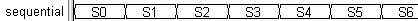```"""Counts upwards from start by step with prepended prefix"""
prefix = 'S'
start = 0
step = 1

index = int((currentT - startT)/deltaT) - 1
value = start + step*index
return prefix + str(value)

```
 Phone: 540-953-3390 | Email: Sales Office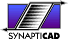COPYRIGHT 2019, SYNAPTICAD SALES, INC., ALL RIGHTS RESERVED.